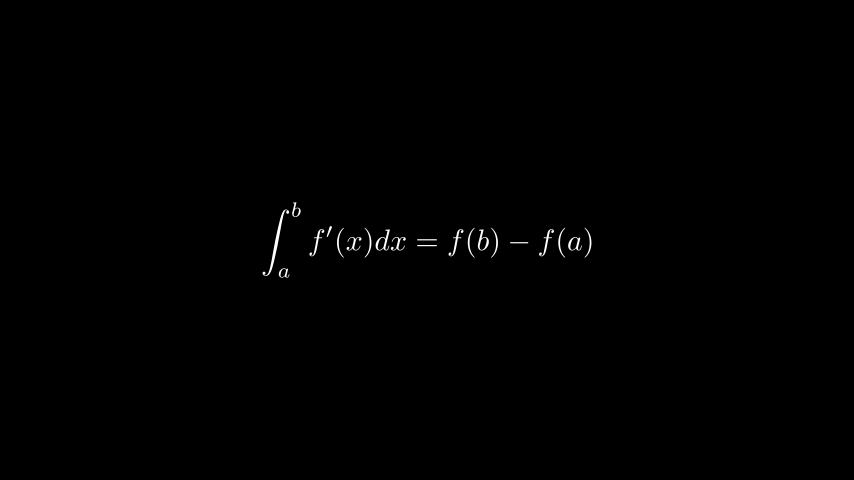# MathTex#

Qualified name: manim.mobject.text.tex\_mobject.MathTex

class MathTex(*tex_strings, arg_separator=' ', substrings_to_isolate=None, tex_to_color_map=None, tex_environment='align*', **kwargs)[source]#

A string compiled with LaTeX in math mode.

Examples

Example: Formulafrom manim import *

class Formula(Scene):
def construct(self):
t = MathTex(r"\int_a^b f'(x) dx = f(b)- f(a)")

class Formula(Scene):
def construct(self):
t = MathTex(r"\int_a^b f'(x) dx = f(b)- f(a)")



Tests

Check that creating a MathTex works:

>>> MathTex('a^2 + b^2 = c^2')
MathTex('a^2 + b^2 = c^2')


Check that double brace group splitting works correctly:

>>> t1 = MathTex('{{ a }} + {{ b }} = {{ c }}')
>>> len(t1.submobjects)
5
>>> t2 = MathTex(r"\frac{1}{a+b\sqrt{2}}")
>>> len(t2.submobjects)
1


Methods

 get_part_by_tex get_parts_by_tex index_of_part index_of_part_by_tex set_color_by_tex set_color_by_tex_to_color_map set_opacity_by_tex Sets the opacity of the tex specified. sort_alphabetically

Attributes

 animate Used to animate the application of any method of self. animation_overrides color depth The depth of the mobject. fill_color If there are multiple colors (for gradient) this returns the first one font_size The font size of the tex mobject. hash_seed A unique hash representing the result of the generated mobject points. height The height of the mobject. n_points_per_curve sheen_factor stroke_color width The width of the mobject.
Parameters:
• arg_separator (str) –

• substrings_to_isolate (Iterable[str] | None) –

• tex_to_color_map (dict[str, ManimColor]) –

• tex_environment (str) –

_break_up_by_substrings()[source]#

Reorganize existing submobjects one layer deeper based on the structure of tex_strings (as a list of tex_strings)

_original__init__(*tex_strings, arg_separator=' ', substrings_to_isolate=None, tex_to_color_map=None, tex_environment='align*', **kwargs)#

Initialize self. See help(type(self)) for accurate signature.

Parameters:
• arg_separator (str) –

• substrings_to_isolate (Optional[Iterable[str]]) –

• tex_to_color_map (dict[str, manim.utils.color.core.ManimColor]) –

• tex_environment (str) –

set_opacity_by_tex(tex, opacity=0.5, remaining_opacity=None, **kwargs)[source]#

Sets the opacity of the tex specified. If ‘remaining_opacity’ is specified, then the remaining tex will be set to that opacity.

Parameters:
• tex (str) – The tex to set the opacity of.

• opacity (float) – Default 0.5. The opacity to set the tex to

• remaining_opacity (float) – Default None. The opacity to set the remaining tex to. If None, then the remaining tex will not be changed